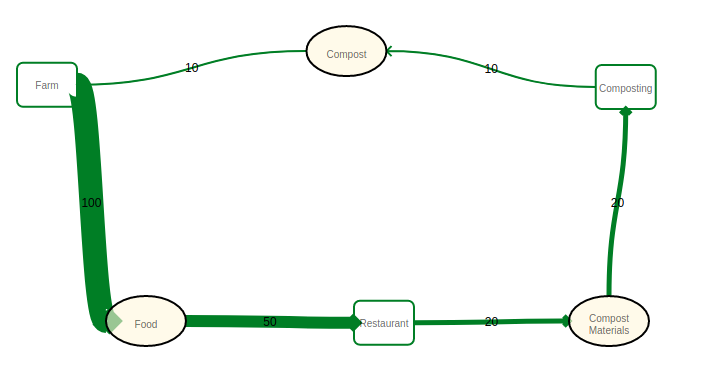#### Howdy, Stranger!

It looks like you're new here. If you want to get involved, click one of these buttons!

Options

# Chapter 1 Example 1.33 cyclic ordering

In the example elements 11, 12, 22, and 21 form a cycle of >= relations. I wonder whether there are such cycles at all with strict > relation? For me a cycle like this is possible only if 11=12=22=21=11, if someone could come up with an example of 11 > 12 > 22 > 21 > 11, that would be mind blown :)

• Options
1.

The thing about your question is that we've been using $$\leq$$ as the symbol for total orders, partial orders, and preorders. We also haven't really been using <, so I'm not completely sure what your question is. The thing you mentioned about the cycle implying that 11=12=22=21=11 is definitely true for partial orders, where all cycles must be trivial. The only difference in the definitions of partial order and preorder is that a preorder allows non-trivial cycles.

Comment Source:The thing about your question is that we've been using \$$\leq\$$ as the symbol for total orders, partial orders, and preorders. We also haven't really been using <, so I'm not completely sure what your question is. The thing you mentioned about the cycle implying that 11=12=22=21=11 is definitely true for partial orders, where all cycles must be trivial. The only difference in the definitions of partial order and preorder is that a preorder allows non-trivial cycles.
• Options
2.
edited April 2018

Yes, as #Joseph suggested here the question goes about preorders, and the particular cycle in the example 1.33. I can readily imagine systems, which are strictly bigger from each other, actually example 1.2 and other examples show systems (partitions) which are partitioned by < relation ((+, *, #) < ((+, *), #). But all such systems form DAGs (directed acyclic graphs). In example 1.33 we were presented with a cycle, and my imagination only allows cycles if all systems forming a cycle are strictly equal. So I wondered whether there exists an (practical) example of such a cyclic preorder where while 1 < 2, 2 < 3, but 3 < 1?

Comment Source:Yes, as #Joseph suggested here the question goes about preorders, and the particular cycle in the example 1.33. I can readily imagine systems, which are strictly bigger from each other, actually example 1.2 and other examples show systems (partitions) which are partitioned by < relation ((+, *, #) < ((+, *), #). But all such systems form DAGs (directed acyclic graphs). In example 1.33 we were presented with a cycle, and my imagination only allows cycles if all systems forming a cycle are strictly equal. So I wondered whether there exists an (practical) example of such a cyclic preorder where while 1 < 2, 2 < 3, but 3 < 1?
• Options
3.

I wondered whether there exists an (practical) example of such a cyclic preorder where while 1 < 2, 2 < 3, but 3 < 1?

Is this such an example with 6 elements, where 1 < 2, 2 < 3, 3 < 4, 4 < 5, 5 < 6, 6 < 1, or did I misunderstand?Comment Source:> I wondered whether there exists an (practical) example of such a cyclic preorder where while 1 < 2, 2 < 3, but 3 < 1? Is this such an example with 6 elements, where 1 < 2, 2 < 3, 3 < 4, 4 < 5, 5 < 6, 6 < 1, or did I misunderstand? ![compost cycle](https://user-images.githubusercontent.com/117439/38198435-767d2560-3652-11e8-978f-93fbd2ee8ae0.png)
• Options
4.
edited April 2018

Generalizing the point made in #3 . If we use preorders to model a cyclical process in time there are many examples. e.g.Comment Source:Generalizing the point made in #3 . If we use preorders to model a cyclical process in time there are many examples. e.g. ![four stroke engine](https://upload.wikimedia.org/wikipedia/commons/d/dc/4StrokeEngine_Ortho_3D_Small.gif) 
• Options
5.
edited April 2018

@Bob, thanks for #3! This is quite a vivid example, indeed this is a cycle, and each time adding additional processing/energy we get something "bigger". @Fredrick, thanks for #4, that's also a cool remark about time, now I'm starting to understand all these better!

Comment Source:@Bob, thanks for #3! This is quite a vivid example, indeed this is a cycle, and each time adding additional processing/energy we get something "bigger". @Fredrick, thanks for #4, that's also a cool remark about time, now I'm starting to understand all these better!
• Options
6.

@Igor Kulieshov: Just a heads up, # at the start of a line creates a headline. This forum uses a markdown variant for comments. @ (similar to twitter and others) is the way to reference other people (though it doesn't understand spaces in names, unfortunately).

# h1

## h2

Comment Source:@Igor Kulieshov: Just a heads up, # at the start of a line creates a headline. This forum uses a markdown variant for comments. @ (similar to twitter and others) is the way to reference other people (though it doesn't understand spaces in names, unfortunately). # h1 ## h2
• Options
7.
edited April 2018

Thanks for the tip, @Jared.

Comment Source:Thanks for the tip, @Jared.
• Options
8.

You can mention a user via a markdown link on their username on their forum comment, like Igor Kulieshov. I have no idea if they get a notification, though.

You can mention their comment the same way using the link on the time on the comment,, like Igor's comment 7.

Comment Source:You can mention a user via a markdown link on their username on their forum comment, like [Igor Kulieshov](https://forum.azimuthproject.org/profile/1923/Igor%20Kulieshov). I have no idea if they get a notification, though. You can mention their comment the same way using the link on the time on the comment,, like [Igor's comment 7](https://forum.azimuthproject.org/discussion/comment/16474/#Comment_16474).
• Options
9.
edited April 2018

Thanks Bob Haugen, it is a helpful suggestion!

Comment Source:Thanks [Bob Haugen](https://forum.azimuthproject.org/profile/1711/Bob%20Haugen), it is a [helpful suggestion](https://forum.azimuthproject.org/discussion/comment/16488/#Comment_16488)!